# Comparing Numbers

There are lots of times when it is good to be able to decide whether one number is bigger, smaller or equal to another. For example, when you go shopping, you might want to work out which one of two toys is the cheaper. You'll be looking for the one with the smaller price. If you want to go on a ride at an amusement park, you might need to be able to decide whether your height is bigger than the minimum allowed height for a ride. When you're playing a card game with a friend, you might need to be able to decide whether you both have equal numbers of cards.

In mathematics, we use special symbols to show whether one number is bigger than, smaller than or equal to another number. Here is a list of these signs, their names, and some examples of their use.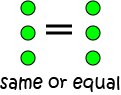We use the equals sign to show that two values are the same, or equal to each other.

For example, $1 + 1 = 2$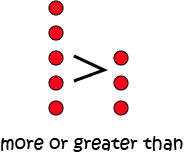We use the greater than sign to show that the value on the left is bigger than the value on the right.

For example, $5 > 3$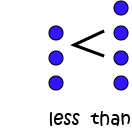We use the less than symbol to show that the value on the left is smaller than the value on the right.

For example, $3 ## The Less Than and Greater Than Signs It can be difficult to remember which way around the less than and greater than symbols go. Just remember that the wide end of each symbol points to the bigger number, and the small end of each symbol points to the smaller number. For the greater than symbol, BIG \(>$ small .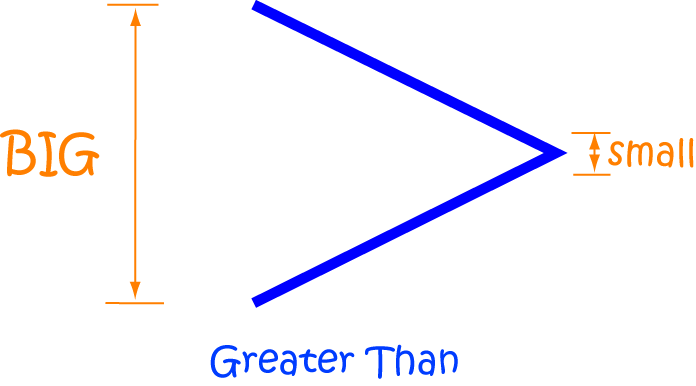For the less than symbol, small $.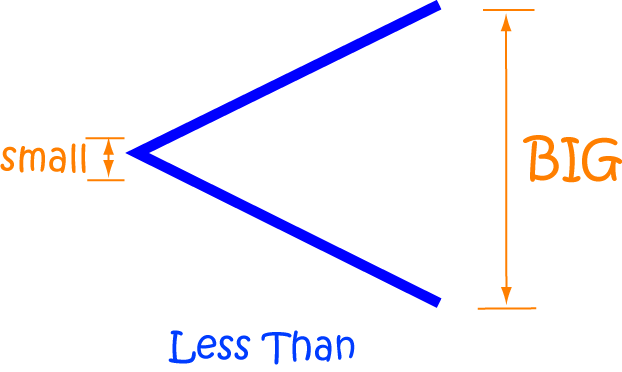## Comparing Numbers from 0 to 10 When we compare numbers from 0 to 10, just think that the number you say first when you count is the smaller one. So, for example, \(3$ is less than $7$ because we say $3$ first when we are counting. So, we would write $3 3$. Finally two numbers are equal if they have the same value, so we can write $7 = 7$.

We can also use the number line to compare numbers from $0$ to $10$. The left-most number on the number line is the one that is smaller.

For example, \(2### Description

This mini book covers the core of Math for Foundation, Grade 1 and Grade 2 mathematics including

1. Numbers
3. Subtraction
4. Division
5. Algebra
6. Geometry
7. Data
8. Estimation
9. Probability/Chance
10. Measurement
11. Time
12. Money
13. and much more

This material is provided free of cost for Parent looking for some tricks for their Prekinder, Kinder, Prep, Year 1 and Year 2 children

### Learning Objectives

These lessons are for kids aged 4-8 with the core objective to expose their brains to concepts of addition, subtraction, division, algebra and much more.

Author: Subject Coach
You must be logged in as Student to ask a Question.